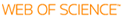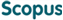### BROWSE

#### Related ResearcherJeong, Won-Ki
High-performance Visual Computing Lab
Research Interests
• Visualization, image processing, machine learning, parallel computing

Curvature-based anisotropic geodesic distance computation for parametric and implicit surfaces

Cited 3 times inCited 5 times inTitle
Curvature-based anisotropic geodesic distance computation for parametric and implicit surfaces
Author
Keywords
Anisotropy; Geodesic; H-J equation; Normal curvature; Parametric and implicit surface; Tensor
Issue Date
2009-08
Publisher
SPRINGER
Citation
VISUAL COMPUTER, v.25, no.8, pp.743 - 755
Abstract
Distribution of geometric features varies with direction, including, for example, normal curvature. In this paper, this characteristic of shape is used to define a new anisotropic geodesic (AG) distance for both parametric and implicit surfaces. Local distance (LD) from a point is defined as a function of both the point and a unit tangent plane directions, and a total distance is defined as an integral of that local distance. The AG distance between points on the surface is the minimum total distance between them. The path between the points that attains the minimum is called the anisotropic geodesic path. Minimization of total distance to attain the AG distance is performed by associating the LD function with a tensor speed function that controls wave propagation in the convex Hamilton-Jacobi (H-J) equation solver. We present new distance metrics for both parametric and implicit surfaces based on the curvature tensor. In order to solve for the implicit AG, a bounded 3D H-J equation solver was developed. We present a second metric for the AG distance, a difference curvature tensor, for parametric surfaces. Some properties of both new AG distances are presented, including parameterization invariance. This AG path differs from the usual geodesic in that minimal path, i.e., lowest cost path, roughly speaking, minimizes an integral of curvature along the curve. Then, the effectiveness of the proposed AG distances as shape discriminators is demonstrated in several applications, including surface segmentation and partial shape matching.
URI
DOI
10.1007/s00371-009-0362-0
ISSN
0178-2789
Appears in Collections:
EE_Journal Papers
Files in This Item: